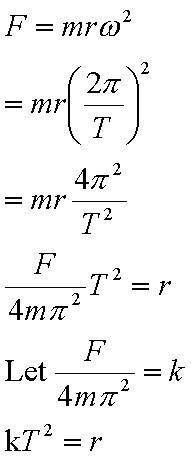# In Centripetal Motion

How is the Period proportional to the Radius. (The Period being how long it takes for 1 full revolution of an object)

I am also assuming there is a tension force.

it looks the square of the period is proportional to the radius, rather.That's what gets me stumped though =/.

The teacher says he is going to put in the test "Show that the radius is proportional to the Radius".

What does it mean to be proportional. (It means they have a relationship I guess) But what would you write down for an answer asking if something is proportional to something else.

If the radius is changed does the Period change?

for a variable to be proportional to another, it implies a relationship of

A = kB (or B = pA)

where A and B are the related variables, and k or p is the constant depending on how you write the equation. thus they can be related as a straight linear line.

the radius does change when the period changes, but that is more of a statement like 'the radius increases when the period is longer', note that this statement does not really have any quantifiable mathematical relationship.